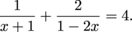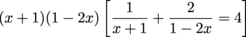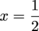Study Guide

Sometimes you'll meet an equation that you think you don't know, and you'll start up a conversation. After a few minutes of talking, though, it finally hits you—this is just like a quadratic equation. You feel super embarrassed and you try to act like you knew it the whole time, but the equation already noticed. If you want to avoid this awkward situation, pay attention to our tips.### Sample Problem

Solve the equationSome equations dedicate themselves to a rigorous regimen of diet and exercise. This tends to make them a little bottom-heavy. They're fractions.

To solve this kind of equation, the first step is to clear out the fractions by multiplying everything by the least common denominator(1 – 2x) + 2(x + 1) = 4(x + 1)(1 – 2x

1 – 2x + 2x + 2 = 4(x – 2x2 + 1 – 2x

3 = 4x – 8x2 + 4 – 8x

8x2 + 4x – 1 = 0

Aw, we just wrecked their diet. We didn't think a single fudge brownie was going to ruin their figure like that. Anyway, from this point everything works like a quadratic equation. Activate the quadratic formula.Remember, though, that our original equation had fractions. When looking for solutions, we want to be sure to avoid division by zero. So, we need to make sure that x = -1 andaren't included in our set of solutions. Neither of our answers is one of the forbidden solutions, so we're okay. Now we double-check our answers.The first answer checks out. Round two.Complete success. Total victory. Correct answer.

### Sample Problem

Solve the equation x4 – 3x2 – 4 = 0.

This guy sure doesn't look quadratic. However, a little bit of lateral thinking shows that the equation actually has the form a(variable)2 + b(variable) + c. For this problem, (variable) = x2. Let's give (variable) a new name, u. We can rewrite the equation as:

(x2)2 – 3(x2) – 4 = 0

u2 – 3u – 4 = 0

This is clearly quadratic. And factorable.

(u – 4)(u + 1) = 0

However, the answer is not u = 4 and u = -1. Our answer needs to be in terms of x, so let's substitute it back into our answers.

u = x2 = 4 and = x2 = -1

So, x = ±2 and x = ±i. Unlike equations that start off being quadratic, this particular kind can have more than two solutions. Check it out by substituting them into the original equation (with x, not the one with u).

f(±2) = (±2)4 – 3(±2)2 – 4 = 16 – 12 – 4 = 0

fi) = (±i)4 – 3(±i)2 – 4 = 1 – 3(-1) – 4 = 0

The real nasty disguises, though, are the ones like x2/3 + x1/3 + 1, which has u = x1/3. Sometimes it takes a bit of imagination to see how a particular function can be made quadratic. So get creative. Maybe pretend it's a unicorn or something.